# Topic 5 Electricity and Magnetism IB PHYSICS Electric

• Slides: 16Topic 5: Electricity and Magnetism. IB PHYSICS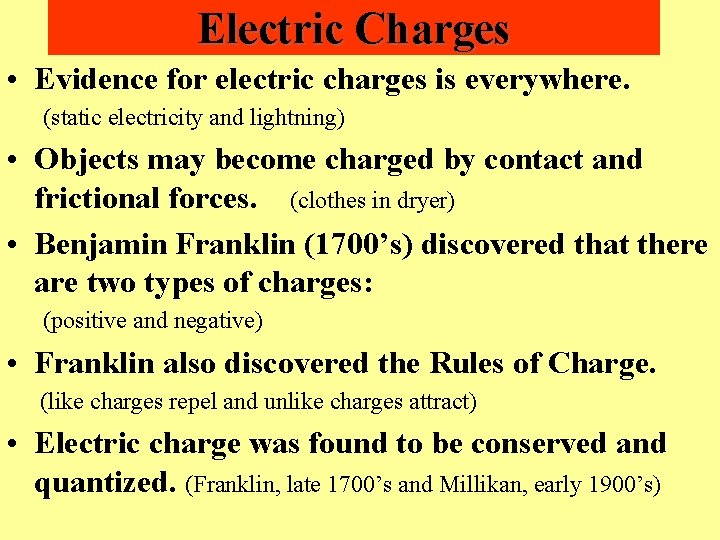Electric Charges • Evidence for electric charges is everywhere. (static electricity and lightning) • Objects may become charged by contact and frictional forces. (clothes in dryer) • Benjamin Franklin (1700’s) discovered that there are two types of charges: (positive and negative) • Franklin also discovered the Rules of Charge. (like charges repel and unlike charges attract) • Electric charge was found to be conserved and quantized. (Franklin, late 1700’s and Millikan, early 1900’s)Classes of Materials • CONDUCTORS are materials in which charges may move freely (Cu, Hg, Au, Al, Fe). • INSULATORS are materials in which charges cannot move freely (rubber, plastic, wood, glass). • SEMICONDUCTORS are materials in which charges may move under some conditions (e. g. Si, Gd, Ge, Y).Charles Coulomb measured the force between charged objects. Later, the unit of charge, the Coulomb, C, was named after him. 1 C = 6. 25 x 1018 electrons, which is very large. We usually have m. C, μC, n. C, and p. C. F=k· (q 1∙q 2) r 2 Coulomb’s Constant Charles Coulomb (1736 -1806) France k = 8. 99 x 109 N∙m 2/C 2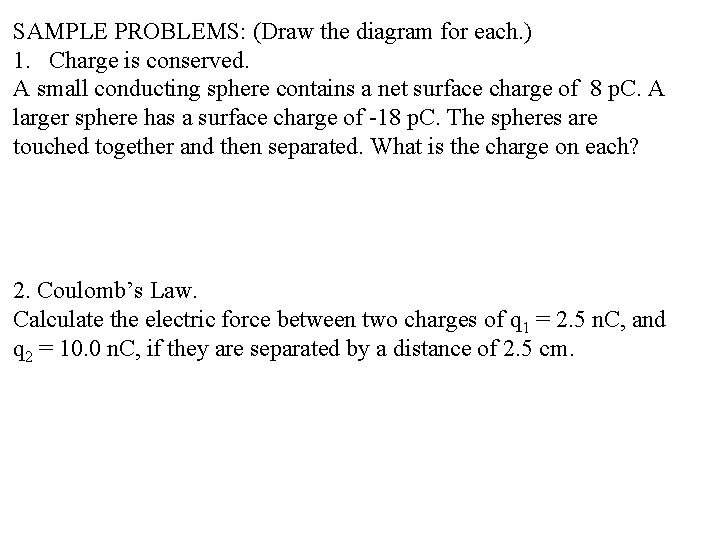SAMPLE PROBLEMS: (Draw the diagram for each. ) 1. Charge is conserved. A small conducting sphere contains a net surface charge of 8 p. C. A larger sphere has a surface charge of -18 p. C. The spheres are touched together and then separated. What is the charge on each? 2. Coulomb’s Law. Calculate the electric force between two charges of q 1 = 2. 5 n. C, and q 2 = 10. 0 n. C, if they are separated by a distance of 2. 5 cm.Benjamin Franklin (1706 -1790), USA, determined that there are only 2 types of charge. Rubber Rod - Negative Glass Rod - Positive Unlike Charges Attract.A charge in space determines an electric field. Electric field lines are lines of force.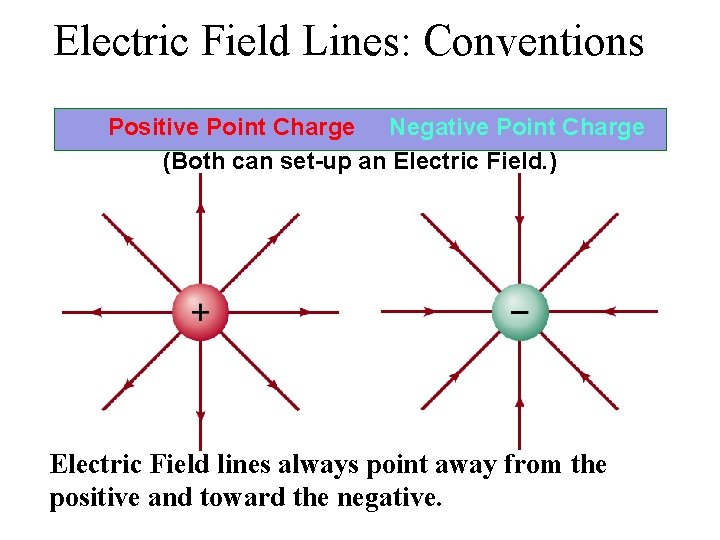Electric Field Lines: Conventions Positive Point Charge Negative Point Charge (Both can set-up an Electric Field. ) Electric Field lines always point away from the positive and toward the negative.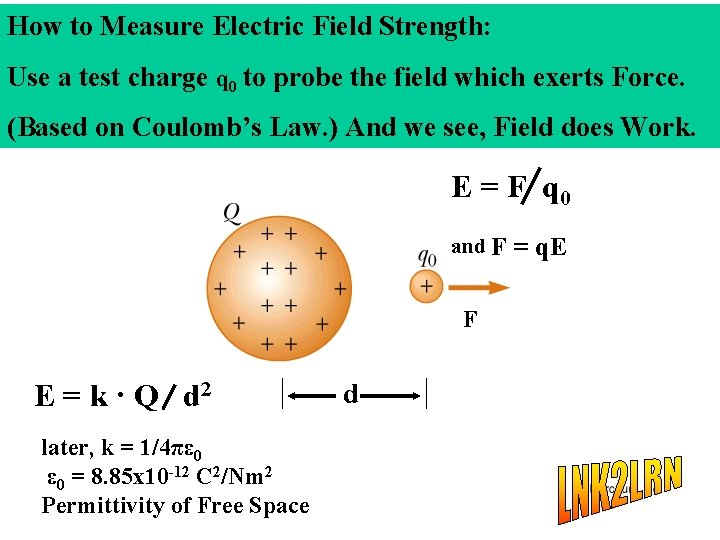How to Measure Electric Field Strength: Use a test charge q 0 to probe the field which exerts Force. (Based on Coulomb’s Law. ) And we see, Field does Work. E = F q 0 and F F E = k · Q d 2 later, k = 1/4πε 0 = 8. 85 x 10 -12 C 2/Nm 2 Permittivity of Free Space d = q. E• Electric charge is quantized. The elementary unit of charge is the charge on one electron or one proton: • 1 e = 1. 602 x 10 -19 C Robert Millikan (1868 -1953) In 1900, while a professor at the University of Chicago. Famous for the “Oil-drop” Experiment. Millikan’s Oil-Drop Experiment (Diagram the forces on a drop. )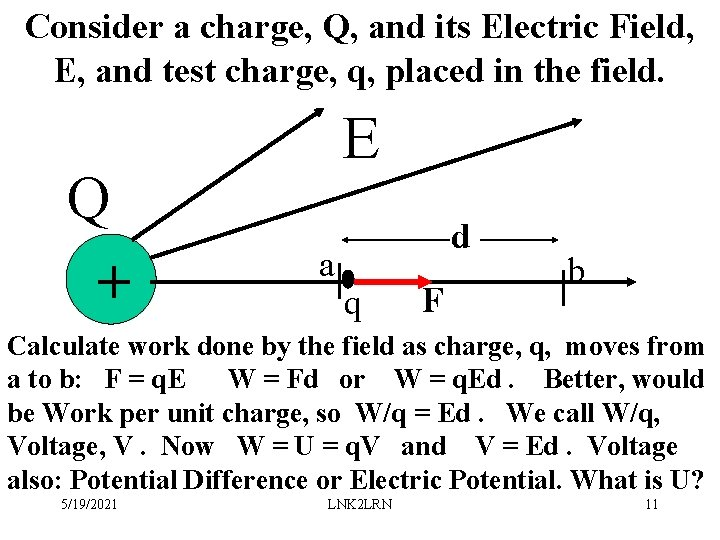Consider a charge, Q, and its Electric Field, E, and test charge, q, placed in the field. Q + E a| q d F b | Calculate work done by the field as charge, q, moves from a to b: F = q. E W = Fd or W = q. Ed. Better, would be Work per unit charge, so W/q = Ed. We call W/q, Voltage, V. Now W = U = q. V and V = Ed. Voltage also: Potential Difference or Electric Potential. What is U? 5/19/2021 LNK 2 LRN 11Ex: What is the speed of a proton accelerated from rest by a voltage of 4. 0 k. V? ( proton mass = 1. 67 x 10 -27 kg, charge = 1. 6 x 10 -19 C ) a. 1. 1 x 106 m/s b. 9. 8 x 105 m/s c. 8. 8 x 105 m/s Ex: To recharge a 12 -V battery, a battery charger must move a charge of 3. 6 x 105 C from the negative to the positive terminal. What amount of work is done by the battery charger? How many kilowatt-hours is this? 1 Kwh costs \$. 13, how much to charge the battery? Ans: 4. 32 x 106 J 1. 2 k. Wh \$. 0156Consider a conducting wire in an Electric Field: Electric Current, I: I = n∙A∙vd∙q Defined as: I = q/ t Unit: C/s or Ampere, Amp, A Named after: Andre Ampere France(1775 -1836).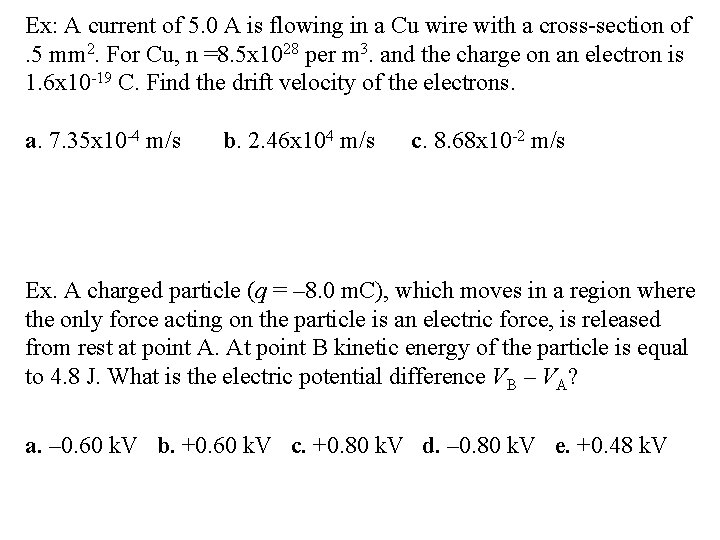Ex: A current of 5. 0 A is flowing in a Cu wire with a cross-section of. 5 mm 2. For Cu, n =8. 5 x 1028 per m 3. and the charge on an electron is 1. 6 x 10 -19 C. Find the drift velocity of the electrons. a. 7. 35 x 10 -4 m/s b. 2. 46 x 104 m/s c. 8. 68 x 10 -2 m/s Ex. A charged particle (q = – 8. 0 m. C), which moves in a region where the only force acting on the particle is an electric force, is released from rest at point A. At point B kinetic energy of the particle is equal to 4. 8 J. What is the electric potential difference VB – VA? a. – 0. 60 k. V b. +0. 60 k. V c. +0. 80 k. V d. – 0. 80 k. V e. +0. 48 k. V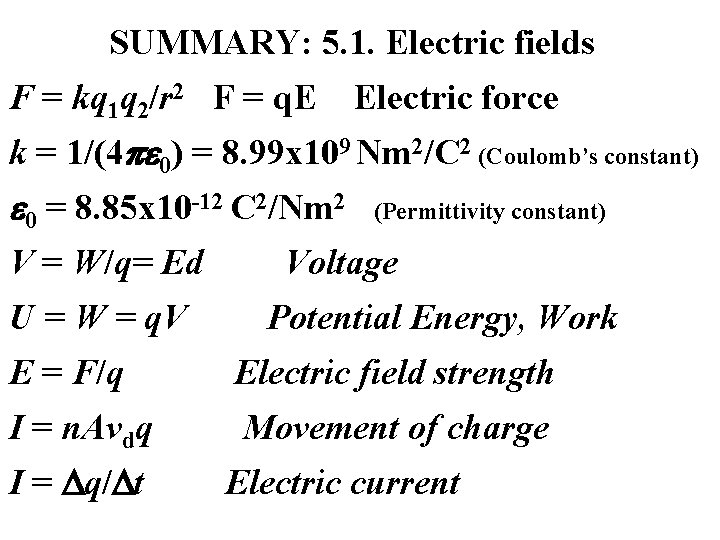SUMMARY: 5. 1. Electric fields F = kq 1 q 2/r 2 F = q. E Electric force k = 1/(4 0) = 8. 99 x 109 Nm 2/C 2 (Coulomb’s constant) 0 = 8. 85 x 10 -12 C 2/Nm 2 V = W/q= Ed U = W = q. V (Permittivity constant) Voltage Potential Energy, Work E = F/q Electric field strength I = n. Avdq Movement of charge I = q/ t Electric current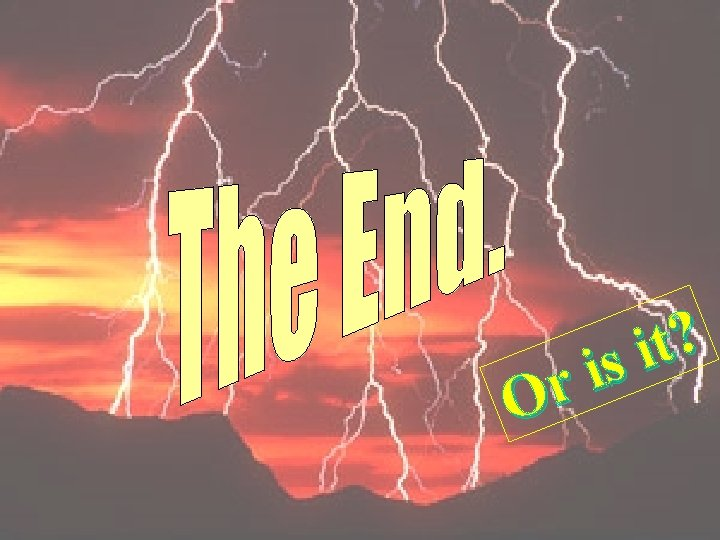r O ? t i is